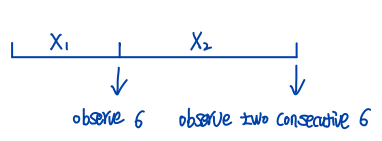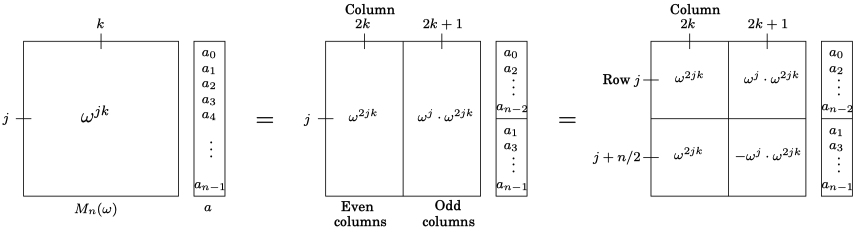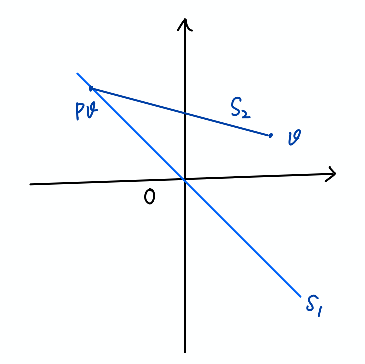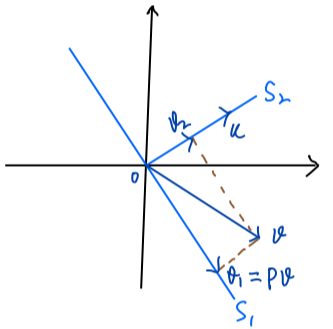This note collects the fundamental concepts, theorems or interesting facts that come into mind in my daily learning.

## Analysis & Probability

#### Limit superior and limit inferior

For a collection of set $$\{E_n\}$$,

$\limsup E_n = \bigcap_{k=1}^\infty \bigcup_{n=k}^\infty E_n,$ $\liminf E_n = \bigcup_{k=1}^\infty \bigcap_{n=k}^\infty E_n.$

$$\limsup$$ on set collection means union starting from infinity $$\bigcup_{n \geq N}^\infty$$ and $$\liminf$$ means intersection starting from infinity $$\bigcap_{n \geq N}^\infty$$.

For a sequence of $$\{x_n\}$$,

$\limsup x_n = \inf_{k \geq 1} \sup_{n \geq k} x_n,$ $\liminf x_n = \sup_{k \geq 1} \inf_{n \geq k} x_n.$

#### Cantor set

Let $$F_0$$ be the interval $$[0, 1]$$. We remove the open interval $$(\frac{1}{3}, \frac{2}{3})$$ from $$F_0$$ and denote the remaining closed set by $$F_1$$. Then we remove the middle interval of length $$\left(\frac{1}{3}\right)^2$$ from each of the two intervals of $$F_1$$ and denote the remaining closed set by $$F_2$$ and so forth as the Figure Cantor Set shown.Figure Cantor Set

Note that we can scale $$F_0$$ by $$\frac{1}{3}$$ to get the left part of $$F_1$$ and then shift the left part by $$\frac{2}{3}$$ to get the right part, that is,

$F_1 = \frac{1}{3} F_0 \bigcup \left(\frac{1}{3} F_0 + \frac{2}{3}\right).$

Similarly, if we scale $$\left[0, \frac{1}{3}\right]$$ and $$\left[\frac{2}{3}, 1\right]$$ by $$\frac{1}{3}$$ we could map them to intervals $$\left[0, \frac{1}{9}\right]$$ and $$\left[\frac{2}{9}, \frac{1}{3}\right]$$, respectively. The two intervals are exactly the left part of $$F_2$$ and we can shift them by $$\frac{2}{3}$$ to get the right part of $$F_2$$, that is,

$F_2 = \frac{1}{3} F_1 \bigcup \left(\frac{1}{3} F_1 + \frac{2}{3}\right).$

Note that the left part of $$F_2$$ is still ranging from $$0$$ to $$\frac{1}{3}$$ and if we scale it by $$\frac{1}{3}$$ we map these intervals into $$\left[0, \frac{1}{9}\right]$$ again. Also, the right part of $$F_2$$ will be mapped into $$\left[\frac{2}{9}, \frac{1}{3}\right]$$ by scaling $$\frac{1}{3}$$. This implies,

$F_n = \frac{1}{3} F_{n-1} \bigcup \left(\frac{1}{3} F_{n-1} + \frac{2}{3}\right),$

holds for $$n = 1, 2, \ldots$$.

The Cantor set is defined as $$F = \bigcap_{n=0}^{\infty} F_n$$. If we represent all numbers in $$[0, 1]$$ in the triadic system, that is, $$\frac{1}{3} a_1 + \frac{1}{3^2} a_2 + \ldots$$ where $$a_n \in \{0, 1, 2 \}$$. Each $$x \in [0, 1]$$ can be represented by a sequence of $$a_1, a_2, \ldots$$, and

\begin{aligned} 0 &= 0, 0, \ldots\\ 1 &= 2, 2, \ldots \end{aligned}

For a $$x = a_1, a_2, \ldots$$ we can interpret the operations $$g$$ of the following

\begin{aligned} \frac{1}{3} &\longrightarrow 0, a_1, a_2, \ldots\\ \frac{1}{3}x + \frac{2}{3} &\longrightarrow 2, a_1, a_2, \ldots \end{aligned}

as prepending 0 and 2 to the sequence $$a_n$$, respectively.

Starting from sequences $$0, 0, \ldots$$, $$2, 2, \ldots$$ and transforming sequences using the above two operations we could generate all possible sequences consisting of 0 and 2. In other words,

$F \stackrel{g}{\longrightarrow} \text{all possible sequences of 0, 2}.$

However, the set of such sequences has the power of the continuum, which can be observed by replacing 2 to 1 and the set corresponds to a binary representation of all numbers in $$[0, 1]$$. Hence, given any $$x \in [0, 1]$$ its preimage in $$F$$ is unique. This implies that $$F$$ has cardinal number not less than the cardinal number of the continuum.

#### Convergence in probability and distribution

A sequence of random variables $$X_1, X_2, \ldots,$$ converge in probability to a random variable $$X$$, that is, $$X_n \stackrel{p}{\rightarrow} X$$, if

$\lim_{n \rightarrow \infty} \mathbb{P} (\vert X_n - X \vert \geq \epsilon) = 0 \quad \text{for all } \epsilon > 0.$

A sequence of random variables $$X_1, X_2, \ldots,$$ converge in distribution to a random variable $$X$$, that is, $$X_n \stackrel{d}{\rightarrow} X$$, if

$\lim_{n \rightarrow \infty} F_{X_n}(x) = F_X(x),$

for all $$x$$ at which $$F_X(x)$$ is continuous.

Example. Let $$X_1, X_2, \ldots,$$ be a sequence of random variable such that

$X_n \sim \mathrm{Binomial}(n, \frac{\lambda}{n}) \quad \text{for } n \in \mathbb{N}, n > \lambda > 0,$

then $$X_n$$ converges in distribution to $$\mathrm{Poisson}(\lambda)$$.

#### NTU bus arrival problem

Suppose Bus 179 arrives every 5 minutes, Bus 199 arrives every 20 minutes and you arrive bus station randomly, what is the probability of 199 arrives first?

Let $$x$$ and $$y$$ be the arrival time of 199 and 179 in a time interval $$[0, 20]$$, $$A$$ be the event of 199 arriving first. By the assumption, the event of 179 arriving must happen within the first 5 minutes therefore $$A \Longleftrightarrow 0 < x < y < 5$$ and

$\mathbb{P}(A) = \int_0^5 \int_x^5 \frac{1}{5} dy \frac{1}{20} dx = \frac{1}{8}.$

#### Dice rolling problem

Let $$X$$ be the number of steps taken to observe two consecutive 6 when rolling a fair dice, what is its distribution?Figure Dice Rolling

Since we need to roll at least two times to observe two consecutive 6, $$X-1$$ is a geometric distribution. Let $$X_1$$ be the number of steps taken to see the first 6 and $$X_2$$ be the number of steps taken to see two consecutive 6 after we have observed the first 6, and $$A$$ be the event of observing 6 in the next step when 6 is observed in the present step. Then, $$X = X_1 + X_2$$ and

\begin{aligned} \mathbb{E}[X] &= \mathbb{E}[X_1] + \mathbb{E}[X_2],\\ \mathbb{E}[X_1] &= 6,\\ \mathbb{E}[X_2] &= \mathbb{E}[X_2 \vert A = 1] \mathbb{P}(A = 1) + \mathbb{E}[X_2 \vert A = 0] \mathbb{P}(A = 0)\\ &= 1 \times \frac{5}{6} + (\mathbb{E}[X] + 1) \times \frac{1}{6}. \end{aligned}

Solving the equation, we obtain $$\mathbb{E}[X] = 42$$. Hence, $$Y = X-1$$ is a geometric distribution with $$\mathbb{E}[Y] = 41$$ or success probability $$p = 1/41$$,

$\mathbb{P}(X = k) = \mathbb{P}(Y = k - 1) = (1-p)^{k-2} p, \quad k = 2, 3, \ldots$

#### Coupon collector problem

When we have collected $$k$$ distinct coupons, the probability of getting a new coupon is $$\frac{n-k}{n}$$. Clearly, the number of steps $$X_k$$ required to take to get the new one follows a geometric distribution with success probability $$\frac{n-k}{n}$$ and $$\mathbb{E}X_k = \frac{n}{n - k}.$$

Hence, the expected total number of steps needed to collect all coupons is

\begin{aligned} \mathbb{E}\left[\sum_{k=0}^{n-1} X_k\right] &= \sum_{k=0}^{n-1}\mathbb{E}[X_k]\\ &= \sum_{k=0}^{n-1} \frac{n}{n-k} = \sum_{k=1}^{n} \frac{n}{k} \\ &= n\left(1 + \frac{1}{2} + \ldots + \frac{1}{n}\right) \approx n \log n. \end{aligned}

## Linear Algebra & Functional

#### Inner product in complex space

Suppose $$V$$ is a vector space over the field $$\mathbb{C}$$ and for $$v, {w} \in V$$, the inner product is denoted by $$\langle v, w \rangle$$. In analysis, the inner product is defined to be linear in the first argument and conjugate-linear in the second argument, that is,

\begin{aligned} \langle \lambda v, w \rangle &= \lambda \langle v, w \rangle\\ \langle v, \lambda w \rangle &= \bar{\lambda} \langle v, w \rangle. \end{aligned}

However, in physics, in particular, quantum mechanics, the conjugate-linearity is defined for the first argument and the linearity is for the second argument,

\begin{aligned} \langle \lambda v, w \rangle &= \bar{\lambda} \langle v, w \rangle\\ \langle v, \lambda w \rangle &= \lambda \langle v, w \rangle. \end{aligned}

The results given by the two definitions differ by a conjugate operation since inner product satisfies conjugate symmetry.

In both definitions, the properties we present for innner product in matrix-calculus hold by replacing transpose with conjugate transpose,

\begin{align} \langle AC, BD \rangle &= \langle B^* AC, D \rangle = \langle C, A^* BD \rangle \\ &= \langle A C D^*, B \rangle = \langle A, B D C^* \rangle. \end{align}

#### Matrix norm

1-norm.

\begin{aligned} \| A \|_1 &= \sup_{\| x \|_1 = 1} \| A x \|_1 = \sup_{\| x \|_1 = 1} \| \sum_{j=1}^n x_j a_j \|_1 \\ &\leq \sup_{\| x \|_1 = 1} \sum_{j}\| x_j a_j \|_1 \leq \max_{1 \leq j \leq n} \| a_j \|_1 \end{aligned}

where $$a_j$$ is the $$j$$-column of $$A$$ and the upper bound is attained when $$x$$ put all energy in the column with the largest 1-norm.

Infinity norm.

\begin{aligned} \| A \|_\infty &= \sup_{\| x \|_\infty = 1} \| A x \|_\infty \\ &= \sup_{\| x \|_\infty = 1} \max_{1 \leq i \leq m} \vert a_i^* x \vert = \max_{1 \leq i \leq m} \sup_{\| x \|_\infty = 1} \vert a_i^* x \vert\\ &\leq \max_{1 \leq i \leq m} \sum_{j=1}^n |a_{ij}| = \max_{1 \leq i \leq m} \|a_i\|_1 \end{aligned}

where $$a_i$$ is the conjugate transpose of $$i$$-th row of $$A$$ and the upper bound can be attained by setting $$x_j = e^{-i\theta_j}$$ if $$\bar{a}_{ij} = r_j e^{i\theta_j}$$ (actually $$x_j$$ can be any value on the unit circle). We can also apply Hölder’s inequality in the second line to obtain the result, that is,

$\sup_{\| x \|_\infty = 1} \vert a_i^* x \vert \leq \|a_i\|_1 \|x\|_\infty = \|a_i\|_1.$

#### Polynomial representation and FFT

A polynomial of degree $$n-1$$ can be represented by $$n$$ coefficient $$c_0, c_1, \ldots, c_{n-1}$$ or its evaluated values at $$n$$ distinct points.

$\left[ \begin{array}{c} p(1)\\ p(x_1)\\ p(x_2)\\ \vdots\\ p(x_{n-1}) \end{array} \right] = \left[ \begin{array}{lllll} 1& 1& 1& \ldots& 1\\ 1& x_1& x_1^2&\ldots &x_1^{n-1}\\ 1& x_2& x_2^2&\ldots &x_2^{n-1}\\ \vdots&\vdots & \vdots& &\vdots\\ 1& x_{n-1}& x_{n-1}^2 & & x_{n-1}^{n-1} \end{array} \right] \left[ \begin{array}{c} c_0\\ c_1\\ c_2\\ \vdots\\ c_{n-1} \end{array} \right]$

Given two polynomials

\begin{aligned} p_1(x) &= a_0 + a_1 x + \ldots + a_{n-1} x^{n-1}, \\ p_2(x) &= b_0 + b_1 x + \ldots + b_{n-1} x^{n-1}. \end{aligned}

The product of $$p_1$$ and $$p_2$$ is a polynomial of $$2n-2$$ degree with $$2n-1$$ coefficients $$c_0, c_1, \ldots, c_{2n-2}$$ and $$c_k = \sum_{i=1}^k a_i b_{k-i}$$. In other words, $$\mathbf{c} = \mathbf{a} \circledast \mathbf{b}$$, the convolution of $$a$$ and $$b$$.

The convolution operation of two length $$n$$ vectors is of complexity $$O(n^2)$$. However, if we could represent $$p_1, p_2$$ using their value representations, we only need to do elementwise multiplication of their values evaluated at $$2n-1$$ distinct points and the result uniquely determines the polynomial $$p_1(x) \cdot p_2(x)$$. In this case, we need to pad $$a$$ and $$b$$ with zeros to be length of $$2n-1$$ like $$[0, \ldots, 0, a_0, \ldots, a_{n-1}]^\top$$ and $$[0, \ldots, 0, b_0, \ldots, b_{n-1}]^\top$$.

Hence, we could first transform the coefficient representation into the value representation by a linear map, and we can do elementwise product in the value representation to get $$p_1(x) \cdot p_2(x)$$. Then we can get back to the coefficient representation by the inverse linear map. However, the linear map (inverse linear map) itself is very expensive $$O(n^2)$$, so can we do better by carefully choosing the linear map?Fig. Roots of Unity

The FFT (Fast Fourier Transform) choose the $$n$$ distinct points to be $$\omega^0, \omega^1, \ldots, \omega^{n-1}$$ where $$\omega = e^{2 \pi i/n}$$, that is, the $$n$$ unitary roots in the unit circle as shown in the Figure and the linear map is defined by matrix

$\Omega = \frac{1}{\sqrt{n}}\left[\begin{array}{lllll} 1& 1& 1& \ldots& 1\\ 1& \omega^ {1 \cdot 1} & \omega^{1\cdot 2}&\ldots &\omega^{1\cdot (n-1)}\\ 1& \omega^{2 \cdot 1}& \omega^{2 \cdot 2}&\ldots &\omega^{2\cdot (n-1)}\\ \vdots&\vdots & \vdots& &\vdots\\ 1& \omega^{(n-1) \cdot 1}& \omega^{(n-1) \cdot 2} & & \omega^{(n-1)\cdot (n-1)} \end{array} \right]$

where we put a normalization factor $$\frac{1}{\sqrt{n}}$$ such that $$\Omega$$ is a unitary matrix, $$\Omega \Omega^* = I$$.

Suppose $$n$$ is the power of 2, the origin-symmetry pairs $$(\omega^k, \omega^{k + n/2})$$ in the unit circle

$- \omega^k = e^{2\pi i k / n + \pi i} = e^{2\pi i (k + n/2)/n} = \omega^{k + n/2},$

implies $$\omega^{2k} = \omega^{2(k + n/2)}$$. In other words, the square operation will reduce the $$n$$ roots of unity $$\omega^0, \omega^1, \ldots, \omega^{n-1}$$ to $$n/2$$ roots of unity $$\omega^0, \omega^2, \ldots, \omega^{n-1}$$. FFT exploits such symmetry by reusing the intermediate computed results to accelerate the transformation. Specifically, for the given origin-symmetry pair $$(\omega^k, \omega^{k + n/2})$$ we group the polynomial coefficients by odd-even as

$p(x) = \underbrace{(c_0 + c_2 x^2 + \ldots + c^{n-2} x^{n-2})}_{P_e(x)} + x\underbrace{(c_1 + c_3 x^2 + \ldots + c^{n-1} x^{n-2})}_{P_o(x)},$

then $$P_e(\omega^k) = P_e(\omega^{k + n/2}))$$ and $$P_o(\omega^k) = P_o(\omega^{k + n/2}))$$ which leads to

\begin{aligned} P(\omega^k) &= P_e(\omega^k) + \omega^k P_o(\omega^k)\\ P(\omega^{k+n/2}) &= P_e(\omega^k) - \omega^k P_o(\omega^k). \end{aligned}

The choice of $$n$$ roots of unity permit repeating the process recursively, that is, we recast the problem of size $$n$$ as two subproblems of size $$n/2$$, followed by some linear-time arithmetic. The running time is

$T(n) = 2 T(n/2) + O(n),$

which is $$O(n \log n)$$. The whole picture is depicted in the figure.Figure FFT in Matrix Form

#### Unitary matrix

(Proposition.) The absolute value or magnitude of the eigenvalues of unitary matrix are 1.

Proof: Suppose $$Q$$ is unitary matrix and $$Q v = \lambda v$$,

$\|v\|_2^2 = \langle Q v, Q v \rangle = \langle \lambda v, \lambda v \rangle = \bar{\lambda}\lambda \|v\|_2^2 = \vert \lambda \vert^2 \|v\|_2^2$

which concludes $$\vert \lambda \vert^2 = 1$$. □

Remark. From its eigvalues we can see a unitary matrix only rotates or reflects a vector in $$\mathbb{C}$$.

#### Hermitian and Spectral Theorem

(Definition.) Let $$V$$ be a finite-dimensional vector space, and $$A: V \rightarrow V$$ be a linear operator. The adjoint of $$A$$ is the linear operator $$A^*: V \rightarrow V$$ characterized by

$\langle A^* v, w \rangle = \langle v, A w \rangle \quad \forall v, w \in V.$

(Proposition.) The adjoint of $$A^*$$ is $$A$$.

Proof: By the definition of adjoint $$\langle A^{**} v, w \rangle = \langle v, A^{*} w \rangle$$, thus it is sufficient to show $$\langle v, A^{*} w \rangle = \langle A v, w \rangle$$.

\begin{aligned} \langle v, A^{*} w \rangle &= \overline{\langle A^{*} w, v \rangle} \quad \text{(conjugate symmetry)}\\ &= \overline{\langle w, A v \rangle} \quad (\text{definition of } A^*)\\ &= \langle A v, w \rangle. \end{aligned}

Proof is completed. □

(Definition.) A linear operator $$A$$ is called self-adjoint or Hermitian if $$A^* = A$$.

(Proposition.) The eigenvalues of Hermtian are real and the eigenvectors corresponding to different eigenvalues are orthogonal.

Proof: Suppose $$v$$ is the eigenvector corresponds to eigenvalue $$\lambda$$,

\begin{aligned} \lambda \| v \|_2^2 &= \langle v, A v \rangle \\ &= \langle A v, v \rangle \quad (A \text{ is Hermitian})\\ &= \overline{\langle v, A v \rangle} \quad (\text{conjugate symmetry})\\ &= \overline{\lambda \| v \|_2^2} \\ &= \overline{\lambda} \| v \|_2^2, \end{aligned}

thus we conclude $$\lambda$$ is real.

Now suppose $$A v_1 = \lambda_1 v_1$$, $$A v_2 = \lambda_2 v_2$$, and $$\lambda_1 \ne \lambda_2$$,

\begin{aligned} \lambda_1 \lambda_2 \langle v_1, v_2 \rangle &= \langle A v_1, A v_2 \rangle \\ &= \langle v_1, A^* A v_2 \rangle \\ &= \langle v_1, A^2 v_2 \rangle \\ &= \langle v_1, \lambda_2^2 v_2 \rangle \end{aligned} \\ \Rightarrow (\lambda_1 \lambda_2 - \lambda_2^2) \langle v_1, v_2 \rangle = 0 \Rightarrow \langle v_1, v_2 \rangle = 0.

Proof is completed. □

(Spectral Theorem.) The Hermitian operator $$A$$ has a complete set of orthonormal eigenvectors. Each eigenvalue is real.

Proof: ntu-note. □

#### ProjectorFigure Projector

(Proposition.) Suppose $$P$$ is a projector that projects $$v$$ onto $$P v$$ along $$(I-P)v$$. Let $$S_1 = \{P v \mid v \in \mathbb{C}^m\}$$ and $$S_2 = \{(I - P) v \mid v \in \mathbb{C}^m\}$$. Then $$P$$ seperates $$\mathbb{C}^m$$ into two space $$S_1$$ and $$S_2$$ such that $$S_1 \bigcap S_2 = \{0\}$$ and

\begin{aligned} S_1 &= \mathrm{range}(P) = \mathrm{null}(I - P),\\ S_2 &= \mathrm{range}(I - P) = \mathrm{null}(P). \end{aligned}

Proof: We shall prove $$\mathrm{range}(I-P)=\mathrm{null}(P)$$ as follows.

First, for any $$v \in \mathrm{null}(P)$$ we have $$v = v - P v = (I-P)v \in \mathrm{range}(I-P)$$. Hence, $$\mathrm{null}(P) \subseteq \mathrm{range}(I-P)$$.

Next, for any $$(I - P) v \in \mathrm{range}(I - P)$$ we have $$P(I-P)v = (P - P^2) v = 0$$. Thus, $$\mathrm{range}(I-P) \subseteq \mathrm{null}(P)$$.

Now, by writing $$P = I - (I - P)$$ we can obtain $$\mathrm{range}(P) = \mathrm{null}(I - P)$$.

$$\mathrm{null}(P) \bigcap \mathrm{null}(I - P) = \{0\}$$ because for any $$v \in \mathbb{C}^m$$ in both of them satisfies

$v = \underbrace{ v - P v}_{\text{in null}(P)} = \underbrace{ (I - P) v}_{\text{in null}(I - P)} = 0.$

Proof is completed. □

Remark. It should be pointed out that a projector projects the space onto a subspace. In the Figure Projector the hyperplane $$S_1$$ is a subspace since it passes through origin.

(Proposition.) The eigenvalues of the projector is either 0 or 1.

Proof: Suppose $$P$$ is a projector and let $$\lambda$$ be its eigenvalue and $$v$$ be the corresponding eigenvector then

$\begin{array}{lll} P v &= \lambda v &\Rightarrow P^2 v &= \lambda P v \Rightarrow\\ P v &= \lambda^2 v &\Rightarrow \lambda v &= \lambda^2 v \end{array}$

If $$v \ne 0$$ then $$\lambda = 0$$ or $$\lambda = 1$$. □Figure Orthogonal Projector

(Orthogonal Projector.) An orthogonal projector projects the space onto a subspace $$S_1$$ along a subspace $$S_2$$, where $$S_1$$ and $$S_2$$ are orthogonal. Any projector that is hermitian is orthogonal projector.

$v_2 = v - P v = (I - P) v \in \mathrm{range}(I - P) = \mathrm{null}(P) = S_2.$

For $$v_2 \in S_2$$, $$P v_2 = 0$$ since $$S_2 = \mathrm{null}(P)$$.Figure Reflector

(Proposition.) If $$P$$ is an orthogonal projector, $$I - 2P$$ is a unitary matrix.

Proof: It is easy to verify $$(I - 2P)(I - 2P)^* = I$$. □

As the Figure Reflector shown $$(I - 2P)v = (I - P)v - Pv = v_2 - v_1$$, that is, $$I - 2P$$ is a reflector. It is used to construct reflector in Householder QR Factorization.